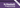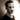Lambda calculus and IThat's some banging purple, right there. Take note, people.

So you wanna learn Haskell, huh? Well, you've come to the right place. Maybe.

This is the first in a series of posts where I try to firm up my understanding of the Haskell programming language by going through the book Haskell Programming from First Principles by Christopher Allen and Julie Moronuki (ISBN: 9781945388033). I'll work my way through the book and write a summary of the most important bits from each chapter as I go along.

## A little bit of background

For a while now, I've been very interested in Haskell and using it whenever I can for my side projects, but I've also been very busy, and haven't had as much time to play with it as I'd wish. I read Learn You a Haskell for Great Good and far too many monad tutorials when I first started out, but since then, it's mostly been a just-in-time sort of process when looking for answers to problems I've come across. So, while I feel I have a basic grasp of the language, some of the more advanced features still escape me.

This book has been on my reading list for a while, but I've not made a serious effort to get through it before. Now, though, I have the time and I have the motivation. It's time to learn Haskell. For real.

Alright, here we go. Deep breaths.

Deep breaths.

## Chapter 1: All you need is Lambda

.... aaaand there's not a single line of Haskell in this whole chapter.

I'm sorry to disappoint you, dear reader, but this chapter is entirely about the theoretical underpinnings of the Haskell language: the lambda calculus. But that doesn't mean that we can't make anything of it! Quite the opposite, in fact.

So put on your math hat and do some light stretches.

...

## Lambda calculus

While a thorough introduction to the lambda calculus is outside the scope of this post (not to mention, not something I'm qualified to do), there are certain concepts that would aid our learning, so let's try a basic introduction.

In the summary of the first chapter, the lambda calculus is defined as "a formal system for expressing programs in terms of abstraction and application." Wow. Such clarity.

Let's see if we can find something more digestible.

Earlier on, the book defines a calculus as a method of calculation or reasoning. In other words, it's a system we can use to solve problems. The lambda calculus is but one process for formalizing one of these methods or systems. In other words, it's a way of thinking about and calculating certain problems and it gives you a set of tools that you can use to solve these.

So why are we looking at the lambda calculus before getting into the code? Well, because functional programming is made up of expressions (values, variables, functions) that model typical mathematical behavior, and understanding how they interact will help us understand how the language works later. Or something.

That's about as much as you'll need to get started. We'll cover anything else as and when it comes up.

## Lambda terms

Let's talk about lambda terms, the three basic components of the lambda calculus. They are:

Variables
A variable is just something you can assign a value to. It has no meaning or value, but only exists as potential input to a function. Simple.
Abstractions
An abstraction is a function. It is a lambda term that has a head (a lambda (𝜆) followed by a variable name) and a body (another expression) and is applied to an argument. An argument is an input value.
Expressions
An expression can be a variable name, an abstraction, or any combination of those two.

A function in the lambda calculus is nothing special. It's just an expression that can be applied to another expression and that returns yet another expression. If this sounds confusing, keep in mind that expressions can be both values and other functions.

A lambda abstraction (that is, a function) looks like this:

\$𝜆x.x\$

where everything from the \$𝜆\$ to the \(.\) (\(𝜆x.\)) is known as the head, and the body is what comes after the \(.\) (\$x\$ in this case). The head binds variables to be used in the body (i.e. function parameters).

Now, an interesting thing to note is that each lambda can only take one argument. Does that mean that in lambda calculus you can only have functions that take a single argument?

Not quite. See, functions that take multiple arguments are actually just nested heads:

\$𝜆x.𝜆y.xy\$

But this is quite verbose, so we'll simplify it by simply writing

\$𝜆xy.xy\$

It might seem trivial but it is a very important property of lambdas, known as currying (after Haskell Curry (no, really)).

This is also what allows for partial application. Because an expression can be partially evaluated, we can also apply it to one value and get a new function back.

For a concrete example, let's say we have a function \$mul\$ that takes two arguments and returns their product:

\$mul=𝜆xy.x*y\$

If we want to create a function that doubles a value, we can do that by applying \$mul\$ to \$2\$:

\$double=mul(2)\$

or, written out as the result of the above expression:

\$double=𝜆y.2*y\$

The \$double\$ function expects one argument and will return the double of whatever it receives and is defined as a partially applied \$mul\$.

## Glossary

How lambda terms interact is probably the most important part of this chapter, but let's have a look at some other terms mentioned in the chapter---mostly because you can use them to sounds smart on internet message boards, but also because they help us understand what we're talking about when we're talking about the lambda calculus.

Alpha equivalence
Two expressions are alpha equivalent if they are the same function, even if they are written with different symbols. The following functions are alpha equivalent:
• \$𝜆a.a\$
• \$𝜆b.b\$
• \$𝜆c.c\$
Application
How we evaluate/reduce lambdas.
Beta normal form
When an expression cannot be beta reduced any further, either because there are no more application to do (no more lambdas), or because there are no free variables to apply the function to.
Beta reduction
Evaluating expressions. This is what happens when you go from the expression

\$𝜆a.a (2)\$ and apply the lambda to the number, thereby ending up with \$2\$

Bound variables
Variables that are declared in the head of a function (y'know, the parameters)
Codomain
The set of possible outputs of a function.
Combinators
A lambda term with no free variables. In other words, all variables are in the head.
Divergence
Any function that never terminates is divergent (hint: recursive functions that never exit, loops of the `while(true)` type).
Domain
The set of possible inputs to a function.
Free variables
Variables that exist in a function body that are not defined in the head, such as the \$y\$ in this expression:

\$𝜆x.xy\$

Lambda
The Greek letter 𝜆. Used to indicate abstractions (functions) and bind variables.
Lambda abstraction
An anonymous function or lambda term. You can think of the head as an abstraction for the body, i.e. something we can put in place of the computation.
Referential transparency (/aka/ purity)
This means that given the same input, a function will always produce the same output.

## Conclusion

While this may seem a strange place to start, I can see the logic in doing it, and getting a better understanding of the lambda calculus is helpful anyway, so I'm fine with starting it this way.

Anyway, there should be more code in the later chapters. Until next time!Thomas Heartman is a developer, writer, speaker, and one of those odd people who enjoy lifting heavy things and putting them back down again. Preferably with others. Doing his best to gain and share as much knowledge as possible.